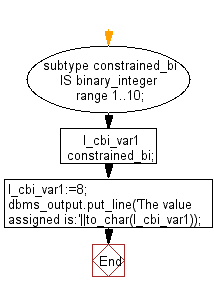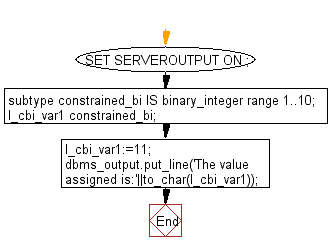﻿ PL/SQL DataType Exercises: Program to show the uses of a constrained subtype - w3resource# PL/SQL DataType: Program to show the uses of a constrained subtype

## PL/SQL DataType: Exercise-8 with Solution

Write a PL/SQL program to show the uses of a constrained subtype.

Sample Solution:

PL/SQL Code:

``````DECLARE
subtype constrained_bi
IS
binary_integer range 1..10;
l_cbi_var1 constrained_bi;
BEGIN
l_cbi_var1:=8;
dbms_output.put_line('The value assigned is:'||to_char(l_cbi_var1));
END;
/
```
```

Sample Output:

```The value assigned is:8
```

Flowchart:In the above example, a subtype is created using the BINARY_INTEGER data type, but with the restriction on the values allowed by using the range keyword. When the variable is assigned with a value outside its range, the unit fails with a VALUE_ERROR. In this example the value is within the range, so no value_error appear.

Now modi the value and see the result-

``````SET SERVEROUTPUT ON ;
DECLARE
subtype constrained_bi
IS
binary_integer range 1..10;
l_cbi_var1 constrained_bi;
BEGIN
l_cbi_var1:=11;
dbms_output.put_line('The value assigned is:'||to_char(l_cbi_var1));
END;
/
```
```

Flowchart:Sample Output:

```DECLARE
*
ERROR at line 1:
ORA-06502: PL/SQL: numeric or value error
ORA-06512: at line 7
```

In this example the value exceeds the range, and the value_error appear.

Improve this sample solution and post your code through Disqus

What is the difficulty level of this exercise?

﻿